Students can Download Maths Chapter 3 Algebra Intext Questions and Answers, Notes Pdf, Samacheer Kalvi 7th Maths Book Solutions Guide Pdf helps you to revise the complete Tamilnadu State Board New Syllabus and score more marks in your examinations.

## Tamilnadu Samacheer Kalvi 7th Maths Solutions Term 2 Chapter 3 Algebra Intext Questions

Exercise 3.1

Try These (Text book Page No. 44)

Question 1.
Observe and complete the following table. First one is done for you.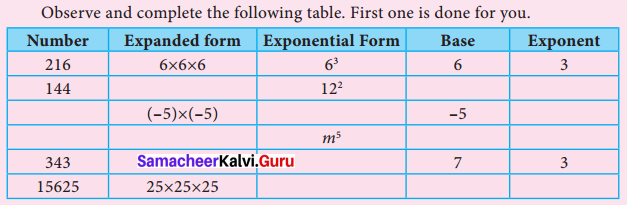Solution: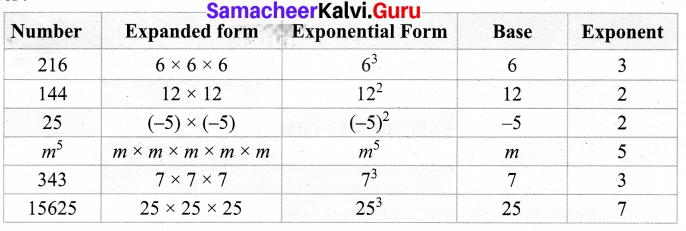Try These (Text book Page No. 46)

Question 1.
Simplify and write the following in exponential form.
1. 23 × 25
2. p2 × P4
3. x6 × x4
4. 31 × 35 × 34
5. (-1)2 × (-1)3 × (-1)5
Solution:
1. 23 × 25 = 23+5 = 28 [since am × an = am+n]

2. p2 × p4 = p2+4 = p6 [since am × an = am+n]

3. x6 × x4 = x6 + 4 = x10 [since am × an = am+n]

4. 31 × 35 × 34 = 31+5 × 34 [since am × an = am+n]
= 36 × 34 [since am × an = am+n]
= 310

5. (-1)2 × (-1)3 × (-1)5
= (-1)2+3 × (-1)5 [Since am × an = am+n]
= (-1)5 × (-1)5
= (-1)5+5 [Since am × an = am+n]
= (-1)10Try These (Text book Page No. 48)

Question 1.
Simply the following.
1. 235 ÷ 232
2. 116 ÷ 113
3. (-5)3 ÷ (-5)2
4. 73 ÷ 73
5. 154 ÷ 15
Solution: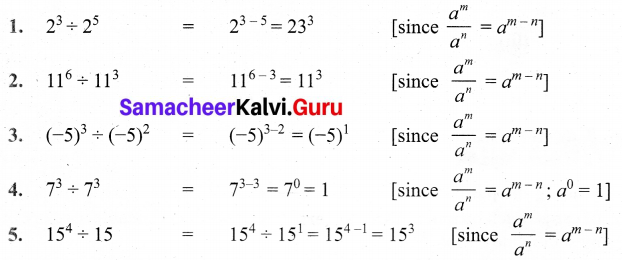Try These (Text book Page No. 48)

Question 1.
Simplify and write the following in exponent form.
1. (32)3
2. [(-5)3]2
3. (206)2
4. (103)5
Solution:
1. (32)3 = 32×3 = 36 [since (am)n = am×n]
2. [(-5)]2 = (-5)3×2 = (-5)6 [since (am)n = am×n]
3. (206)2 = 206×2 = 2012 [since (am)n = am×n]
4. (103)5 = 103×5 = 1015 [since (am)n = am×n]

Question 2.
Express the following exponent numbers using am × bm = (a × b)m.
(i) 52 × 32
(ii) x3 × y3
(iii) 74 × 84
Solution:
(i) 52 × 32 = (5 × 3)2 = 152 [since am × bm = (a × b)m]
(ii) x3 × y3 = (x × y)3 = (x y)3
(iii) 74 × 84 = (7 × 8)4 = 564Question 3.
Simplify the following exponent numbers by using ($$\frac { a }{ b }$$)m = $$\frac{a^{m}}{b^{m}}$$
(i) 53 ÷ 23
(ii) (-2)4 ÷ 34
(iii) 86 ÷ 56
(iv) 63 ÷ (-7)3
Solution:
(i) 53 ÷ 23 = ($$\frac { 5 }{ 2 }$$)3 – [Since $$\frac{a^{m}}{b^{m}}$$ = ($$\frac { a }{ b }$$)m]
(ii) (-2)4 ÷ 34 = ($$\frac { -2 }{ 3 }$$)4
(iii) 86 ÷ 56 = ($$\frac { 8 }{ 6 }$$)6
(iv) 63 ÷ (-7)3 = ($$\frac { 6 }{ -7 }$$)3Exercise 3.2

Try These (Text book Page No. 54)

Question 1.
Find the unit digit of the following exponential numbers:
(i) 10621
(ii) 258
(iii) 3118
(iv) 2010
Solution:
(i) 10621 Unit digit of base 106 is 6 and the power is 21 and is positive.
Thus the unit digit of 10621 is 6.

(ii) 258 Unit digit of base 25 is 5 and the power is 8 and is positive.
Thus the unit digit of 258 is 5.

(iii) 3118 Unit digit of base 31 is 1 and the power 18 and is positive.
Thus the unit digit of 3118 is 1.

(iv) 2010 Unit digit of base 20 is 0 and the power 10 and is positive.
Thus the unit digit of 2010 is 0.

Try These (Text book Page No. 55)

Question 1.
Find the unit digit of the following exponential numbers:
(i) 6411
(ii) 2918
(iii) 7919
(iv) 10432
Solution:
(i) 6411 Unit digit of base 64 is 4 and the power is 11 (odd power).
∴ Unit digit of 6411 is 4.

(ii) 2918 Unit digit of base 29 is 9 and the power is 18 (even power).
Therefore, unit digit of 2918 is 1.

(iii) 7919 Unit digit of base 79 is 9 and the power is 19 (odd power).
Therefore, unit digit of 7919 is 9.

(iv) 10432 Unit digit of base 104 is 4 and the power is 32 (even power).
Therefore, unit digit of 10432 is 6.Exercise 3.3

Try These (Text book Page No. 35)

Question 1.
Complete the following table: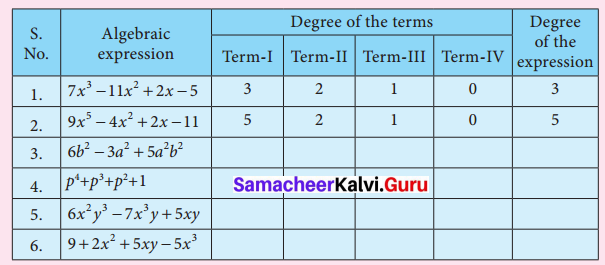Solution: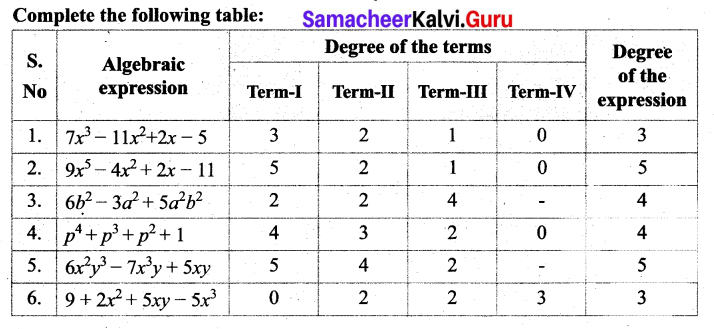Question 2.
Identify the like terms from the following:
(i) 2x2y, 2xy2,3xy2,14x2y, 7yx
(ii) 3x3y2, y3x, y3x2, – y3x, 3y3x
(iii) 11pq, -pq, 11pqr, -11pq,pq
Solution:
(i) 2x2y, 2xy2, 3xy2, 14x2y, 7yx
(a) 2x2y and 14x2y are like terms.
(b) 2xy2 and 3xy2 are like terms.

(ii) 3x3y2, y3x, y3x2, – y3x, 3y3x
(a) y3x, – y3x and 3y3x are like terms.

(iii) 11 pq, -pq, 11pqr , -11 pq, pq
(a) 11 pq, -pq, -pq and pq are like terms.